# Extreme values using INLA

Julian Faraway 21 September 2020

``````library(INLA)
library(brinla)
``````

# Data

Extreme flows in rivers are of special interest since flood defences must be designed with these in mind. The National River Flow Archive provides data about river flows in the United Kingdom. For this example, we consider data on annual maximum flow rates from the River Calder in Cumbria, England from 1973 to 2014:

``````data(calder, package = "brinla")
plot(Flow ~ WaterYear, calder)
``````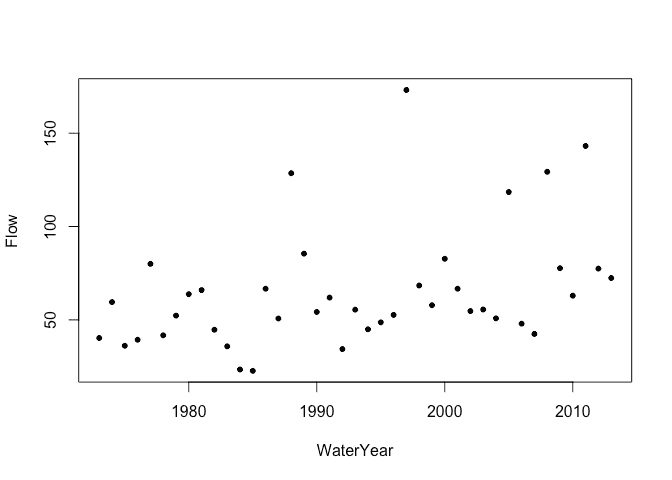Rescale year for convenience:

``````calder\$year = calder\$WaterYear-1973
``````

# Generalized Extreme value distribution

Some scaling is necessary to get the model to fit. Generalized extreme value distributions are notoriously difficult to fit. It is not unusual to see failures to fit which require some tinkering to rectify. The current implementation is marked as experimental so you may experience some difficulties with the fit. We will need the ```control.compute = list(config=TRUE)``` in the computations later in this example.

``````imod <- inla(Flow ~ 1 + year, data = calder, family = "gev", scale = 0.1, control.compute = list(config=TRUE))
imod\$summary.fixed
``````
``````                mean      sd 0.025quant 0.5quant 0.975quant     mode        kld
(Intercept) 36.70809 5.84433    25.1725 36.69416     48.279 36.64147 6.5922e-05
year         0.75959 0.24709     0.2637  0.76249      1.241  0.76952 1.1209e-05
``````

We can see that there is positive linear trend term indicating the peak flows for this river are increasing over time. We can plot the fixed effect posterior for the year:

``````plot(imod\$marginals.fixed\$year, type="l")
``````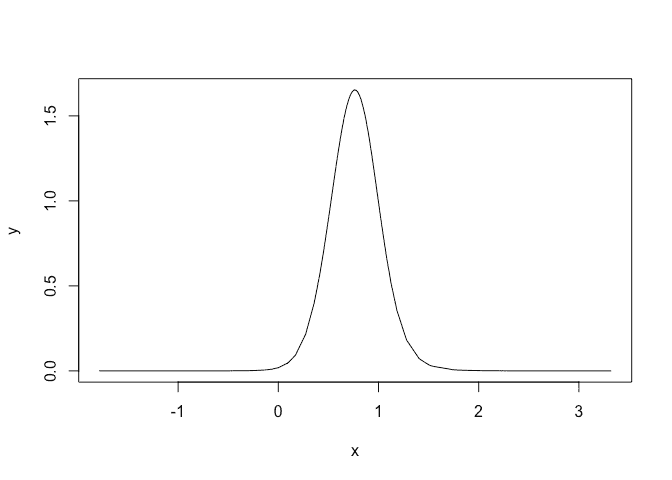We can see that the posterior for the trend in year is concentrated above zero indicating evidence of an increasing trend. Probability that slope is negative is:

``````inla.pmarginal(0, imod\$marginals.fixed\$year)
``````
`````` 0.0020089
``````

Rather small.

Plot the posterior for the precision:

``````plot(imod\$marginals.hyperpar\$`precision for GEV observations`,type="l",xlim=c(0,0.1))
``````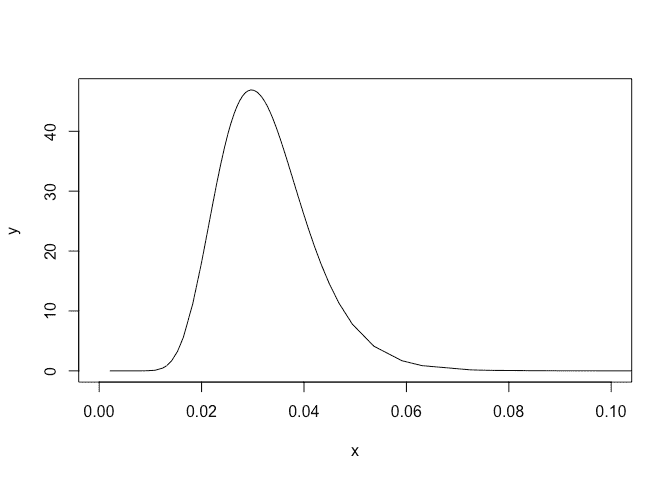or plot that on an SD scale:

``````plot(bri.hyper.sd(imod\$marginals.hyperpar\$`precision for GEV observations`),type="l")
``````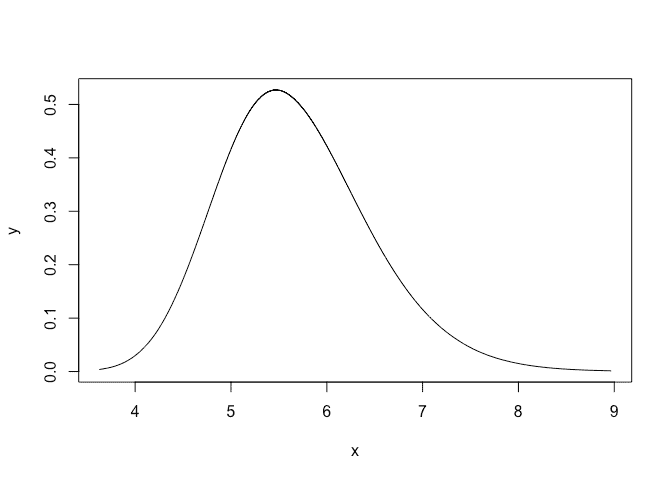Plot the posterior for the tail parameter:

``````plot(imod\$marginals.hyperpar\$`tail parameter for GEV observations`,type="l",xlim=c(-0.2,0.5))
``````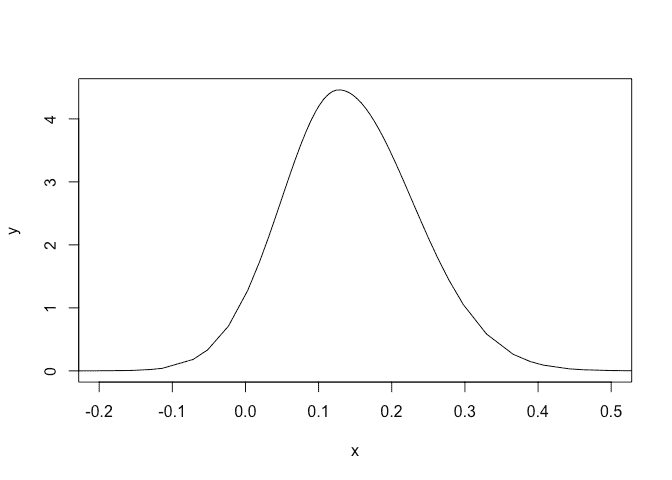A small chance that this is negative.

# Predictions

The maximum flow over the period observation occured in the 1997 water year measuring 173.17 m^3/s. Under our fitted model, what was the probability of observing such a flow (or greater)? This will give us a measure of how unusual this event was. First we need an `R` function to compute P(Y < y) for the generalized extreme value distribution:

``````pgev = function(y,xi,tau,eta,sigma=1){
exp(-(1+xi*sqrt(tau*sigma)*(y-eta))^(-1/xi))
}
``````

Compute probability of observed flow less than this maximum flow in 1997:

``````yr = 1997-1973
maxflow = 173.17
eta = sum(c(1,yr)*imod\$summary.fixed\$mean)
tau = imod\$summary.hyperpar\$mean
xi = imod\$summary.hyperpar\$mean
sigma = 0.1
(pless = pgev(maxflow, xi, tau, eta,sigma))
``````
`````` 0.99113
``````

So probability of exceeding the observed value is:

``````1-pless
``````
`````` 0.0088652
``````

Hydrologists often work with the expected time for the event to occur called the recurrence interval. In this case, the value is:

``````1/(1-pless)
``````
`````` 112.8
``````

Now set year to 2017:

``````yr = 2017-1973
maxflow = 173.17
eta = sum(c(1,yr)*imod\$summary.fixed\$mean)
tau = imod\$summary.hyperpar\$mean
xi = imod\$summary.hyperpar\$mean
sigma = 0.1
pless = pgev(maxflow, xi, tau, eta,sigma)
1/(1-pless)
``````
`````` 71.818
``````

We see that the recurrence interval substantially reduced.

# Credibility interval

Can compute a 95% credibility interval. Need to sample from the full posterior. This is where we need the ```control.compute = list(config=TRUE)``` option.

``````nsamp = 999
postsamp = inla.posterior.sample(nsamp, imod)
pps = t(sapply(postsamp, function(x) c(x\$hyperpar, x\$latent[42:43])))
colnames(pps) <- c("precision","shape","beta0","beta1")
``````

Plot the sampled hyperparameters:

``````plot(pps[,1],pps[,2]*0.01,xlab="Precision",ylab="Shape")
``````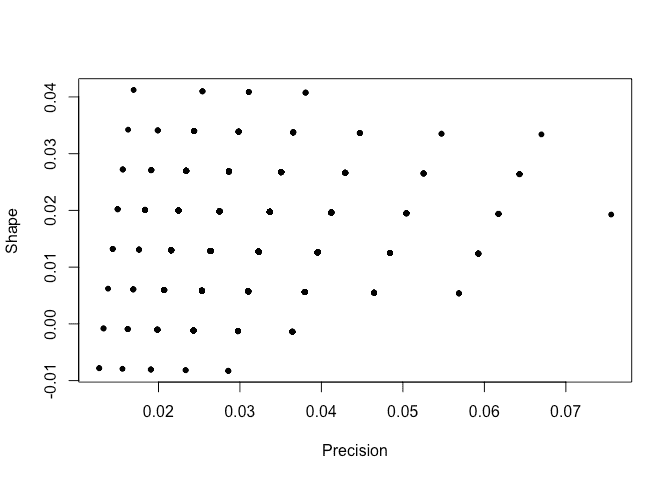We see that the sampled hyperparameters are concentrated on a sparse grid. Here is the sampled posterior for the linear model parameters:

``````plot(pps[,3],pps[,4])
``````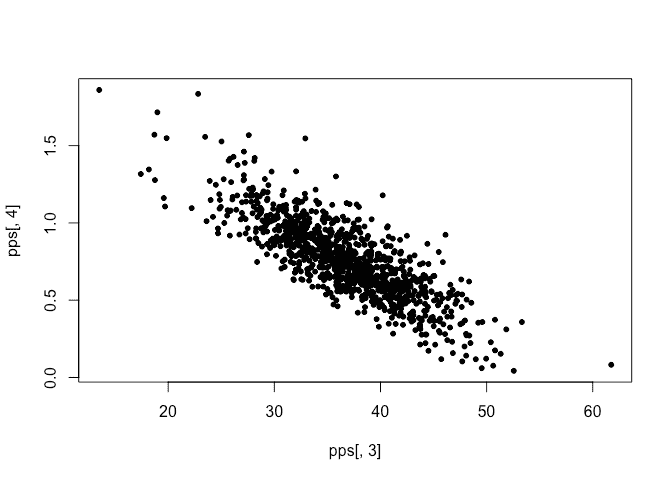No problem with this. We compute the recurrency interval for each sample.

``````sigma = 0.1
maxflow = 173
retp = numeric(nsamp)
for(i in 1:nsamp){
eta = sum(c(1,yr)*pps[i,3:4])
tau = pps[i,1]
xi = 0.01*pps[i,2]
pless = pgev(maxflow, xi, tau, eta,sigma)
retp[i] = 1/(1-pless)
}
``````

From this we can compute the credibility interval:

``````quantile(retp, c(0.025, 0.5, 0.975))
``````
``````    2.5%      50%    97.5%
53.629  246.841 1490.882
``````

This is quite wide. If we want a denser grid for the hyperparameters, we can redo INLA with a smaller step size for the grid. We will get denser coverage but it’s still a grid. This is somewhat undesirable but not as bad as it seems because these are the integration points.

# Package versions

``````sessionInfo()
``````
``````R version 4.0.2 (2020-06-22)
Platform: x86_64-apple-darwin17.0 (64-bit)
Running under: macOS Catalina 10.15.6

Matrix products: default
BLAS:   /Library/Frameworks/R.framework/Versions/4.0/Resources/lib/libRblas.dylib
LAPACK: /Library/Frameworks/R.framework/Versions/4.0/Resources/lib/libRlapack.dylib

locale:
 en_GB.UTF-8/en_GB.UTF-8/en_GB.UTF-8/C/en_GB.UTF-8/en_GB.UTF-8

attached base packages:
 parallel  stats     graphics  grDevices utils     datasets  methods   base

other attached packages:
 brinla_0.1.0  INLA_20.03.17 foreach_1.5.0 sp_1.4-2      Matrix_1.2-18 knitr_1.29

loaded via a namespace (and not attached):
 codetools_0.2-16     lattice_0.20-41      digest_0.6.25        grid_4.0.2           MatrixModels_0.4-1
 magrittr_1.5         evaluate_0.14        rlang_0.4.7          stringi_1.4.6        rmarkdown_2.3
 splines_4.0.2        iterators_1.0.12     tools_4.0.2          stringr_1.4.0        Deriv_4.0.1
 xfun_0.16            yaml_2.2.1           compiler_4.0.2       htmltools_0.5.0.9000
``````